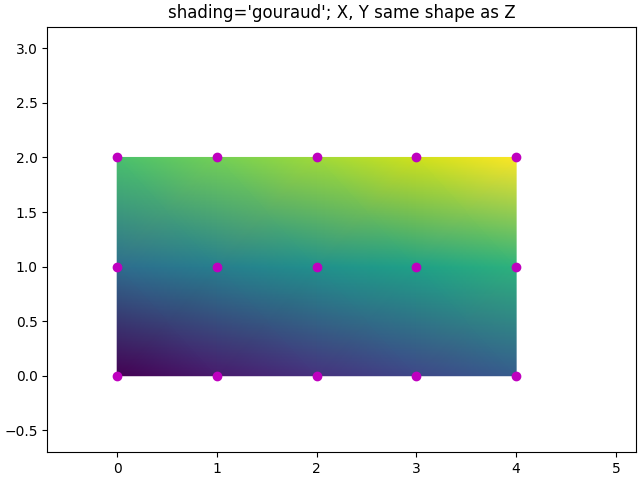axes.Axes.pcolormesh and pcolor have a few options for how grids are laid out and the shading between the grid points.

Generally, if Z has shape (M, N) then the grid X and Y can be specified with either shape (M+1, N+1) or (M, N), depending on the argument for the shading keyword argument. Note that below we specify vectors x as either length N or N+1 and y as length M or M+1, and pcolormesh internally makes the mesh matrices X and Y from the input vectors.

import matplotlib
import matplotlib.pyplot as plt
import numpy as np


The grid specification with the least assumptions is shading='flat' and if the grid is one larger than the data in each dimension, i.e. has shape (M+1, N+1). In that case X and Y specify the corners of quadrilaterals that are colored with the values in Z. Here we specify the edges of the (3, 5) quadrilaterals with X and Y that are (4, 6).

nrows = 3
ncols = 5
Z = np.arange(nrows * ncols).reshape(nrows, ncols)
x = np.arange(ncols + 1)
y = np.arange(nrows + 1)

fig, ax = plt.subplots()
ax.pcolormesh(x, y, Z, shading='flat', vmin=Z.min(), vmax=Z.max())

def _annotate(ax, x, y, title):
# this all gets repeated below:
X, Y = np.meshgrid(x, y)
ax.plot(X.flat, Y.flat, 'o', color='m')
ax.set_xlim(-0.7, 5.2)
ax.set_ylim(-0.7, 3.2)
ax.set_title(title)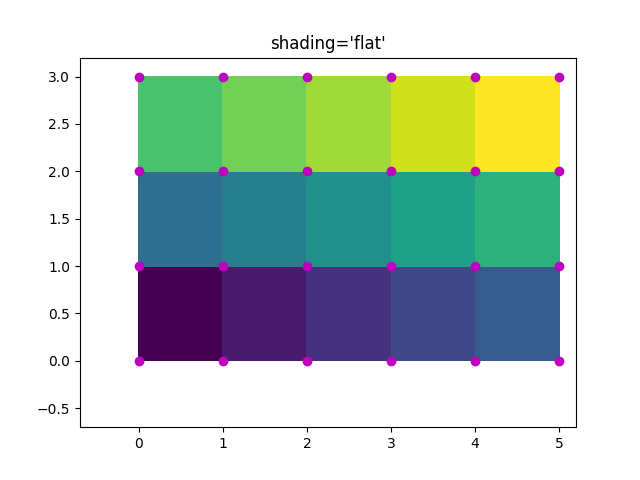## Flat Shading, same shape grid¶

Often, however, data is provided where X and Y match the shape of Z. As of Matplotlib v3.3, shading='flat' is deprecated when this is the case, a warning is raised, and the last row and column of Z are dropped. This dropping of the last row and column is what Matplotlib did silently previous to v3.3, and is compatible with what Matlab does.

x = np.arange(ncols)  # note *not* ncols + 1 as before
y = np.arange(nrows)
fig, ax = plt.subplots()
ax.pcolormesh(x, y, Z, shading='flat', vmin=Z.min(), vmax=Z.max())
_annotate(ax, x, y, "shading='flat': X, Y, C same shape")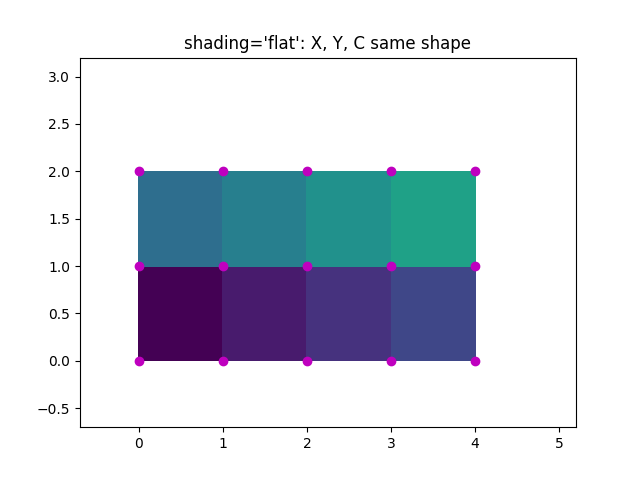Out:

/root/matplotlib/examples/images_contours_and_fields/pcolormesh_grids.py:66: MatplotlibDeprecationWarning: shading='flat' when X and Y have the same dimensions as C is deprecated since 3.3.  Either specify the corners of the quadrilaterals with X and Y, or pass shading='auto', 'nearest' or 'gouraud', or set rcParams['pcolor.shading'].  This will become an error two minor releases later.
ax.pcolormesh(x, y, Z, shading='flat', vmin=Z.min(), vmax=Z.max())


## Nearest Shading, same shape grid¶

Usually, dropping a row and column of data is not what the user means when they make X, Y and Z all the same shape. For this case, Matplotlib allows shading='nearest' and centers the colored quadrilaterals on the grid points.

If a grid that is not the correct shape is passed with shading='nearest' an error is raised.

fig, ax = plt.subplots()
ax.pcolormesh(x, y, Z, shading='nearest', vmin=Z.min(), vmax=Z.max())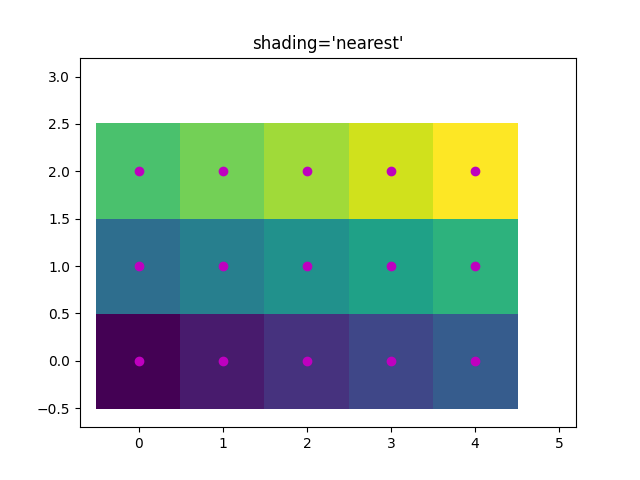It's possible that the user would like the code to automatically choose which to use, in this case shading='auto' will decide whether to use 'flat' or 'nearest' shading based on the shapes of X, Y and Z.

fig, axs = plt.subplots(2, 1, constrained_layout=True)
ax = axs
x = np.arange(ncols)
y = np.arange(nrows)
ax.pcolormesh(x, y, Z, shading='auto', vmin=Z.min(), vmax=Z.max())
_annotate(ax, x, y, "shading='auto'; X, Y, Z: same shape (nearest)")

ax = axs
x = np.arange(ncols + 1)
y = np.arange(nrows + 1)
ax.pcolormesh(x, y, Z, shading='auto', vmin=Z.min(), vmax=Z.max())
_annotate(ax, x, y, "shading='auto'; X, Y one larger than Z (flat)")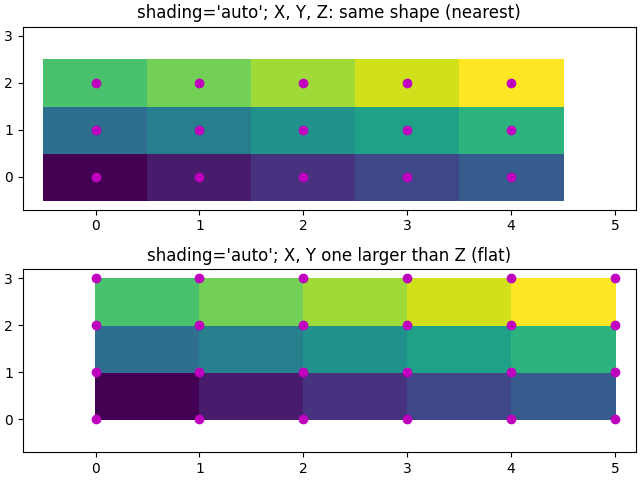fig, ax = plt.subplots(constrained_layout=True)